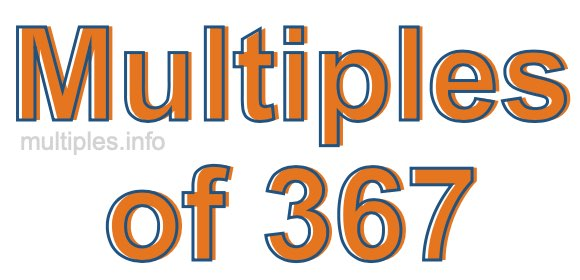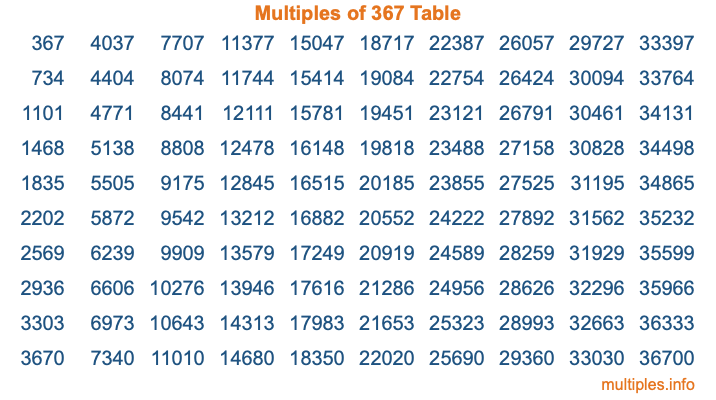Multiples of 367Welcome to the Multiples of 367 page. Here we will first teach you everything you will ever need to know about the multiples of 367, and then give you a study guide summary of everything we taught you to make sure you remember it all. Use this page to look up facts and learn information about the multiples of 367. This page will make you a multiples of three hundred sixty-seven expert!

Definition of Multiples of 367
Multiples of 367 are all the numbers that when divided by 367 equal an integer. Each of the multiples of 367 are called a multiple. A multiple of 367 is created by multiplying 367 by an integer.

Therefore, to create a list of multiples of 367, you start with 1 multiplied by 367, then 2 multiplied by 367, then 3 multiplied by 367, and so on for as long as you want. Thus, the list of the first five multiples of 367 is 367, 734, 1101, 1468, and 1835. To see a larger list of multiples of 367, see the printable image of Multiples of 367 further down on this page. We also have a category where you can choose any nth multiple of 367.

Multiples of 367 Checker
The Multiples of 367 Checker below checks to see if any number of your choice is a multiple of 367. In other words, it checks to see if there is any number (integer) that when multiplied by 367 will equal your number. To do that, we divide your number by 367. If the the quotient is an integer, then your number is a multiple of 367.

Is  a multiple of 367?

Least Common Multiple of 367 and ...
A Least Common Multiple (LCM) is the lowest multiple that two or more numbers have in common. This is also called the smallest common multiple or lowest common multiple and is useful to know when you are adding our subtracting fractions. Enter one or more numbers below (367 is already entered) to find the LCM.

Check out our LCM Calculator if you need more details about the Least Common Multiple or if you need the LCM for different numbers for adding and subtraction fractions.

nth Multiple of 367
As we stated above, 367 is the first multiple of 367, 734 is the second multiple of 367, 1101 is the third multiple of 367, and so on. Enter a number below to find the nth multiple of 367.

th multiple of 367

Multiples of 367 vs Factors of 367
367 is a multiple of 367 and a factor of 367, but that is where the similarities end. All postive multiples of 367 are 367 or greater than 367. All positive factors of 367 are 367 or less than 367.

Below is the beginning list of multiples of 367 and the factors of 367 so you can compare:

Multiples of 367: 367, 734, 1101, 1468, 1835, etc.

Factors of 367: 1, 367

As you can see, the multiples of 367 are all the numbers that you can divide by 367 to get a whole number. The factors of 367, on the other hand, are all the whole numbers that you can multiply by another whole number to get 367.

It's also interesting to note that if a number (x) is a factor of 367, then 367 will also be a multiple of that number (x).

Multiples of 367 vs Divisors of 367
The divisors of 367 are all the integers that 367 can be divided by evenly. Below is a list of the divisors of 367.

Divisors of 367: 1, 367

The interesting thing to note here is that if you take any multiple of 367 and divide it by a divisor of 367, you will see that the quotient is an integer.

Multiples of 367 Table
Below is an image of the first 100 multiples of 367 in a table. The table is in chronological order, column by column. The first column has the first ten multiples of 367, the second column has the next ten multiples of 367, and so on.The Multiples of 367 Table is also referred to as the 367 Times Table or Times Table of 367. You are welcome to print out our table for your studies.

Negative Multiples of 367
Although not often discussed or needed in math, it is worth mentioning that you can make a list of negative multiples of 367 by multiplying 367 by -1, then by -2, then by -3, and so on, to get the following list of negative multiples of 367:

-367, -734, -1101, -1468, -1835, etc.

Multiples of 367 Summary
Below is a summary of important Multiples of 367 facts that we have discussed on this page. To retain the knowledge on this page, we recommend that you read through the summary and explain to yourself or a study partner why they hold true.

There are an infinite number of multiples of 367.

A multiple of 367 divided by 367 will equal a whole number.

367 divided by a factor of 367 equals a divisor of 367.

The nth multiple of 367 is n times 367.

The largest factor of 367 is equal to the first positive multiple of 367.

367 is a multiple of every factor of 367.

367 is a multiple of 367.

A multiple of 367 divided by a divisor of 367 equals an integer.

367 divided by a divisor of 367 equals a factor of 367.

Any integer times 367 will equal a multiple of 367.

Multiples of a Number
Here you can get the multiples of another number, all with the same attention to detail as we did for multiples of 367 on this page.

Multiples of
Multiples of 368
Did you find our page about multiples of three hundred sixty-seven educational? Do you want more knowledge? Check out the multiples of the next number on our list!